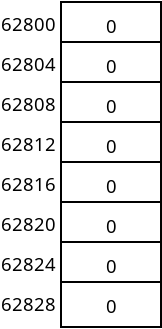# Arrays as a Data Structure

## Overview

Arrays are one of the simplest data structures, and the only one built into Java directly. Arrays can have multiple dimensions and contain a fixed number of elements.

Array elements are stored contiguously in memory which means that the elements take up consecutive memory cells, there are no "gaps" between items.

We will look at arrays as a data structure, and consider how efficient they are at various tasks.

## One-dimensional Arrays

We might create an array of integers as follows:


int[] array = new int;


When an array is created like this, enough space for storing 8 integers is set aside in memory. For instance, if the memory assigned to this array begins at address 62800, then the cells would be organized like this:An array in memory

An integer in Java takes 4 bytes, so each cell is 4 bytes large, and the array takes 32 bytes in total.

We can then access one of the elements in the array using an index:


array = 42;
System.out.println(array);


When the expression array is evaluated, the computer must find the address of array element 3. To do this, it uses the following formula:

$address = start + (size \times index)$

In this example, start is 62800, the index is 3, and the size of each element is 4. That gives us 62812 as the index of array element 3.

There are a couple of important points regarding this:

• Indexing an array is a very fast operation. There is no need to "scan through" elements to find what we need. We can jump right there.
• This only works if the elements are stored together with no gaps, and if all the elements are the same size.
• This is why arrays start at 0. If we put in 1 for the index, then we get an address 4 bytes past the start, which is the second element.
• It's not possible to insert new cells into, or remove cells from the middle of the array.

## Multi-dimensional Arrays

We can also create multi-dimensional arrays, which are arrays of arrays. There is no limit to the number of dimensions of an array that you create. However, high numbers of dimensions are rarely used. Here we will discuss two-dimensional arrays.

A two-dimensional array can be created in Java with:


char[][] grid = new char;


This will create a two-dimensional array with 12 total elements. With a two-dimensional array, we normally think of it as having 3 rows, and 4 columns.

We usually think of two-dimensional arrays as tables. However, computer memory is always flat and one-dimensional. So a two-dimensional array must actually be stored one-dimensionally.

In Java, and in most common programming languages, this is done using a row-major scheme. That means the rows are stored one after the other, as shown in the following figure:A logical view of a 2D array (left) vs. how it is actually laid out in memory (right)

To access the elements of a multi-dimensional array, we must supply an index for every dimension. For instance, we could use the following code to set row 2, column 1 to 'J', as in the figure above:


grid = 'J';


The indexing formula used in this operation is now a little more complicated:

$address = start + (row \times N_{columns}) + (col \times size)$

We again start with whatever the starting address for the array is. Then we add the row we want multiplied by how many columns there are. This is done to "skip over" rows before the one we want. We then multiply the column index multiplied by the size of each element.

In the example above, we'd have 2 for the row, 4 for the number of columns, 1 for the column, and 1 for the size of each element. That gives us 9 bytes past the start of the array, which you can verify on the image above.

## Advantages and Disadvantages of Arrays

Arrays have a number of advantages as a data structure:

• We can jump directly to any element by giving the index.
• They are compact in memory.
• Looping over an array is very efficient.
• They are built into Java and many other languages.

There are disadvantages to arrays too:

• We must specify the size of the array in advance.
• There's no way to append to an array which is full.
• Cells that are not being used will waste space.
• Adding to or removing from the middle of an array is not efficient.

## Program Parameters

Arrays are used in Java for passing parameters to our programs. When you run a program on the command line, you can pass it parameters. For example, if you run the cp command to copy a file, you have to tell it what file to copy and to where. And if you open a file in Vim, you need to tell it which file.

This is done by passing that information as command-line parameters:

$cp MyProgram.java backup/$ vim MyProgram.java


But how can we write a program which accesses these parameters? The answer is in the args parameter to main, which most of the time you have probably just ignored.

For example, the following program prints out all of the command-line parameters that are passed to it:


public class Parameters {
public static void main(String args[]) {
for (int i = 0; i < args.length; i++) {
System.out.println("Argument " + i + " = " + args[i]);
}
}
}


If we run this program like normal, nothing at all happens:

$java Parameters$ java Parameters have some arguments
Argument 0 = have
Argument 1 = some
Argument 2 = arguments


We can use this to pass parameters into our programs that we write.

Copyright © 2021 Ian Finlayson | Licensed under a Creative Commons Attribution 4.0 International License.